## Current Issues

### Numerical Simulation of a Thermosyphon Radiator Used in Electronic Devices

1 School of Energy Science and Engineering , Harbin Institute of Technology , China

J Robot Mech Eng

Article Type : Research Article

### Abstract

The heat dissipation of electronic devices is an important issue. The thermosyphon radiators have high heat dissipation performance, so they are gradually widely used in electronic devices. In this study, a numerical model of the thermosyphon is established. It is observed that simulated temperatures agree well with experimental data in the literature with a relative error no more than 4%. After the numerical model is validated, it is used in the simulation of the thermosyphon radiator. The wall temperature of the condensing section under different thermal load conditions is compared, and the thermal resistance of the condensing section is analyzed. The results show that with the increase of heating and condensing heat flux, the wall temperature fluctuation of the condensing section increases, but very small just about 5K, 6K, 7K, and 9K, respectively. The thermal resistance of the condensing section decreases, indicating that the thermosyphon radiator has a better performance under high heat flux conditions.

Keywords: Thermosyphon Radiator; Phase Change Heat Transfer; Numerical Simulation; Wall Temperature; Thermal Resistance Analysis

# Introduction

Increasing heating power can lead to larger heat flux and bring thermal issues in the design of electronic devices. If heat is not effectively released, the reliability and the lifetime of the electronic devices will decrease seriously. Therefore, heat dissipation is considered an important factor in the design of electronic devices [1,2]. Among all the heat dissipation methods, thermosyphons have the best effect due to their high thermal conductivity, good temperature uniformity and low thermal resistance . The phase change heat transfer process and vapor-liquid two-phase flow of thermosyphons are very complicated and difficult to describe, and it is also difficult to explore the mechanism in detail through experiments . Numerical simulation is an effective method to predict the thermal performance of thermosyphons before conducting experiments, which can reduce research time and cost. Some researchers have adopted different methods to simulate the heat and mass transfer process of thermosyphons. Because the VOF model can clearly capture the vapor-liquid two-phase fluid interface, some researchers use the VOF model to describe the phase change process of the working fluid inside the thermosyphon [6-8]. Alizadehdakhel et al.  modeled a vapor-liquid two-phase flow and the simultaneous evaporation and condensation phenomena in a thermosyphon. They employed VOF model to capture the interface between the phases. It was concluded that Computational Fluid Dynamics (CFD) is a powerful tool for fluid dynamics and thermal design in a thermosyphon. Fadhl et al. [10,11] simulated the details of the two-phase flow and heat transfer characteristic during the start-up and steady-state operation of a thermosyphon by considering the mass transfer time relaxation parameter as a constant in the VOF model, described the heat and mass transfer process of the thermosyphon as water, R134a, and R404a. The predicted temperature profiles showed good agreement with experimental data and the investigated working medium were R134a and R404a. Alammar et al.  used the VOF model to study the influence of the inclination angle and liquid filling rate of the thermosyphon on its heat transfer characteristics during the two-phase flow of the working fluid inside the thermosyphon. Results showed that there was a significant increase in the evaporator temperature at low filling ratio and low inclination angle. The minimum thermal resistance was obtained at filling ratio of 65% and inclination angle of 90°. Schepper et al.  established a phase change model based on the VOF model to realize the visualization of the phase change process of the working fluid inside the thermosyphon. Kuang et al.  used the VOF model to simulate the flow boiling behavior of a separated heat pipe and gave a vapor-liquid two-phase flow pattern distribution map. Wang et al.  designed a heat pipe for a linear focusing solar receiver, using the VOF model to describe the internal vapor-liquid two-phase heat transfer and mass transfer process, and found that a condenser with a certain inclination angle can enhance its heat transfer performance.

The above studies have been numerically simulated for a single thermosyphon, but little has research on the thermosyphon radiator composed of multiple condensation sections and the same evaporation section. To clarify the heat dissipation performance of the radiator under different heat flow power, a numerical model of the thermosyphon is established. After validation, it is used in the simulation calculation of the thermosyphon radiator. Then, the wall temperature and the thermal resistance of the condensing section of the radiator under different heating and condensing heat loads were calculated.

# Mathematical Approach

Governing Equations
Using the VOF (Volume of Fluid) model, the distribution characteristics of the vapor-liquid two-phase composition in the thermosyphon can be realized by solving the phase volume fraction. In the VOF control equation,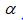is defined as the phase volume fraction in the calculation unit.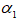and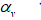represent the liquid volume fraction and the vapor volume fraction in the vapor-liquid two-phase fluid, respectively. The sum of the vapor-liquid two-phase volume fraction in the cell is 1, the expression is as follows: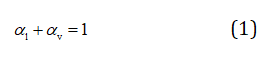To track the vapor-liquid two-phase interface, the continuity equation of VOF is expressed as: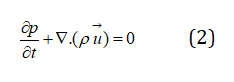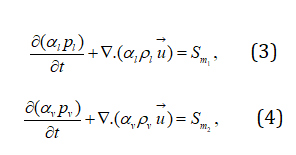where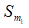and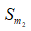are respectively expressed as the mass source terms of the evaporation and condensation process, which are used to calculate the mass transfer of the vapor-liquid two-phase during the evaporation and condensation process. The expressions will be described in the following phase change model. And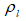and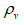respectively represent the density of the liquid phase and the density of the vapor-liquid phase in kg/m3.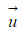Represents the velocity of the vapor-liquid mixed phase in m/s. Momentum equation: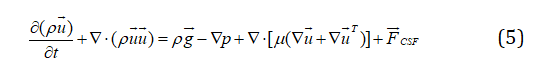On the right side of the momentum equation, the physical meanings of each item in turn are gravity, pressure, friction, and surface tension. In the above formula,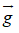represents the acceleration of gravity, in m/s2;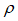represents pressure, in Pa;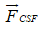represents surface tension, in N/m3. To consider the effect of the surface tension of the vapor-liquid interface in the two-phase fluid (the continuous surface force term in the momentum equation), the continuous surface force model (CSF) proposed by Brackbill et al.  is loaded into the momentum equation. To understand the momentum source term, we conduct the following analysis. Using the divergence theorem, the expression of surface tension can be converted into a volume force to add this volume force to the source term of the momentum equation. If there are only two phases in a control unit, then the following relational expression should be added in :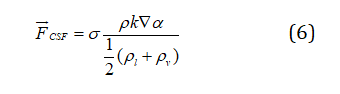In the equation,is the average density calculated by the equation, and the unit is kg/m3. It can be seen from the above equation that the surface tension in the control unit is proportional to the average density of the unit.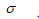Is the surface tension coefficient, the unit is N/m; k is the surface curvature, the unit is 1/m. The expression of surface curvature k is as follows: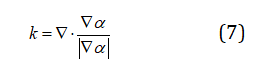The expression of the energy equation for the fluid calculation area is: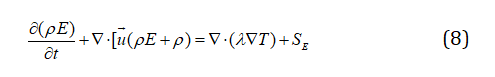SE is the source term of the energy equation, used to calculate the amount of energy transferred in the evaporation and condensation process. E represents energy, the unit is J/kg; T represents the temperature, the unit is K. The density, thermal conductivity, and dynamic viscosity in the above equation are expressed by the volume fraction average method: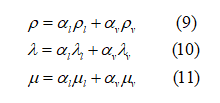The expressions of the two-phase fluid mass and energy source terms in the specific phase change process are shown in Table 1.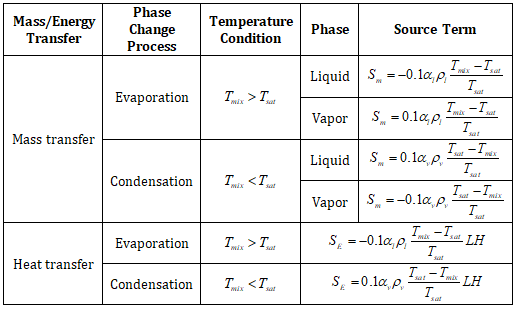Table 1: The source term expression of the phase transition process.

Validation of the Numerical Model

The thermosyphon model in literature  is selected for verification. The model is a 2D model. The length of the evaporation section, the adiabatic section and the condensation section are 100 mm, 100 mm and 150 mm, respectively, the inner diameter is 16.5 mm, the wall thickness is 0.8mm, and the shell material for aluminum. The circle on the left wall represents the arrangement of 12 thermocouples along the height of the thermosyphon to measure the wall temperature of the evaporation section, the adiabatic section and the condensation section respectively, as shown in Figure 1. The working medium filled in the heat pipe is acetone, and its physical parameters are shown in Table 2. The liquid filling rate (the ratio of the liquid volume to the total volume of the heat pipe) is 28.6%. Since the phase change occurs near the wall, the boundary layer grid is encrypted, the first layer is 0.1, the growth rate is 1.1, and the total grid number is 44919, as shown in Figure 2.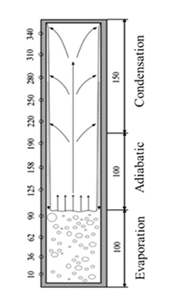Figure 1: Two-dimensional view.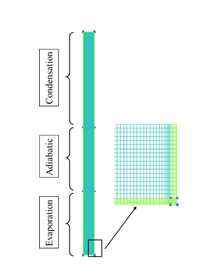Figure 2: Computational grids.

 Physical Property Unit Liquid Phase Value Vapor Phase Value Density kg/m3 748.95 ideal gas law Specific heat capacity J/(kg·K) 2229.3 1567.3 Thermal conductivity W/(m·K) 0.1409 0.014 Viscosity Pa·s 2.3986×10-4 8.3032×10-6 Molecular mass kg/kmol 58.05 Latent heat kJ/kg 501.42 \ Surface tension N/m 0.0188 \

Table 2: Physical properties of acetone.

Figure 3 shows a graph of the vapor-liquid two-phase volume fraction of the thermosyphon changing with time under the condition of 100 W heating powers in the evaporation section. The boiling process and the formation of liquid film can be clearly seen from the figure. The numerical model can simulate the heat and mass transfer of the boiling and condensation reflux well.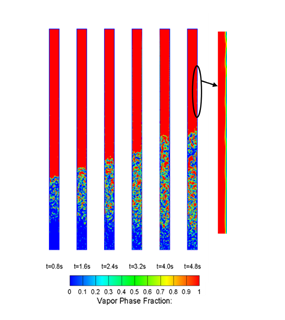Figure 3: The vapor-liquid two-phase volume fraction changes with time.

Figure 4 shows the comparison between the simulation results of the temperature distribution on the outer wall of the thermosyphon along the height direction (0-350 mm) and the experimental results of literature . It can be seen from the figure that all of the numerical results are within the 4% error bar of experimental data, which present a good agreement of numerical data and experimental data. Therefore, the numerical model can be used to simulate the vapor-liquid two-phase flow and heat transfer characteristics.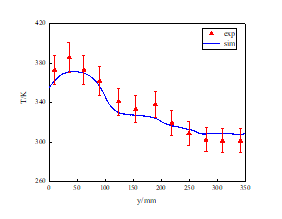Figure 4: Experimental and simulation results of temperature distribution on the outer wall along the height direction.

Physical Model and Grids A 2D geometric model of a thermosyphon radiator with multiple condensation sections is shown in Figure 5. The evaporation section, the adiabatic section and the condensation section are represented by red, black, and blue lines respectively. The blue lines in the figure are named cw_1 to cw_8 from left to right. And the dimensions of each part are marked in the figure (unit: mm). To save the calculation time, and to highlight the calculation of the boiling condensing phase transformation heat of the internal fluid domain and the vapor-liquid two-phase flow, the heat conduction process of the metal shell is no longer calculated. The grid division is shown in Figure 6, the boundary layer is encrypted, the first layer is 0.1, the growth rate is 1.1, and the total number of grids is 80040.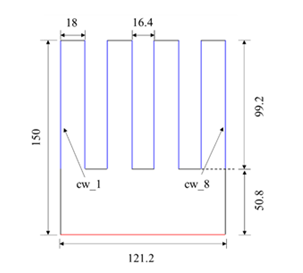Figure 5: Structure and geometry parameters of thermosyphon radiator.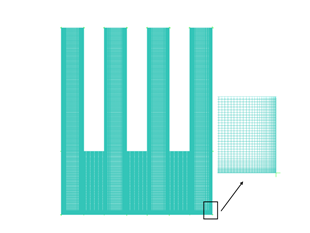Figure 6: Grids of thermosyphon radiator.

Solving Conditions and Numerical Approach

Both the evaporation section and the condensing section of the thermosyphon radiator adopt constant heat flow density conditions, and the adiabatic section is set as an adiabatic wall surface, that is, the heat flow is 0. The initial temperature and pressure of the calculation domain are 329 K and 101.325 kPa, respectively. And the height of the liquid level is 50.8 mm, which is set by the patch function. The heat flux values of the evaporating section and the condensing section are respectively set for 4 working conditions, as shown in Table 3. The energy equation and momentum equation are both discretized in the second-order upwind style, the phase volume fraction term is discretized in the Geo-Reconstruct format, the pressure difference item is discretized in the PRESTO format, and the coupling relationship between velocity and pressure is processed by the SIMPLE method. The residual values of the mass, momentum, and energy equations are controlled at 10-3, 10-6, and 10-6 respectively.

 Cases Heat flux in the evaporation/(W/m2) Heat flux in the condensation (Single wall)/(W/m2) case1 9900 1500 case2 13200 2000 case3 16500 2500 case4 19800 3000

Table 3: Different working conditions.

# Results and Discussion

Wall Temperature Distribution of the Condensation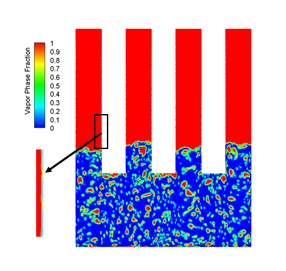Figure 7: Two-phase distribution contour of thermosyphon radiator.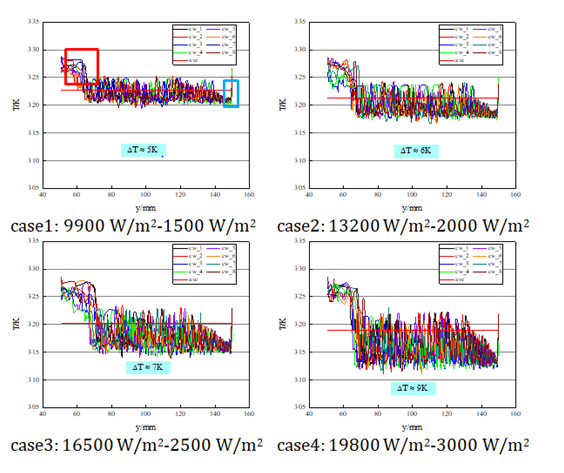Figure 8: Wall temperature distribution of the condensing section under different thermal load conditions.

Thermal Resistance Analysis of the Condensing Section

The comparison of the wall temperature of the condensing section of the thermosyphon radiator under different thermal load conditions is shown in Figure 9. It can be seen from the figure that as the heating and condensing power increases, the temperature of the condensing wall decreases, and the temperature of each wall is slightly different. However, the temperature difference is within 1K, indicating that the radiator has better working temperature uniformity in multiple condensation sections. To further clarify the influence of thermal load conditions on the heat transfer performance of the condensing section, the thermal resistance values of each condensing wall surface under different thermal load conditions were compared, and the results are shown in Figure 10. It can be seen from the figure that as the thermal load increases, the thermal resistance of each condensing wall decreases. This is because although the heat transfer increases, the difference between the temperature of the condensing wall and the working temperature of the working fluid does not change much, so the thermal resistance will decrease as the power increases.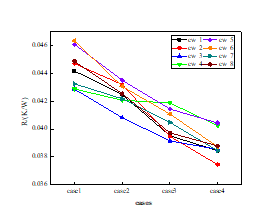Figure 9: Comparison of wall temperature of the condensation section of thermosyphon radiator under different thermal load conditions.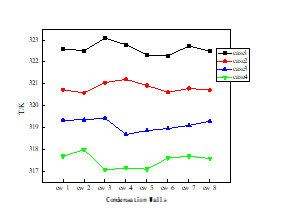Figure 10: Comparison of the thermal resistance of the condensing section of the thermosyphon radiator under different thermal load conditions.

# Conclusion

The VOF model and the phase change model are used to carry out a numerical simulation study on the thermosyphon radiator. The main conclusions from the study are as follows: As the heat load of the evaporation section and the condensation section increases,
1.    The temperature fluctuation range of the condensation section of the thermosyphon radiator becomes larger, but the maximum fluctuation range is no more than 10K.
2.    The wall temperature of each condensing section of the thermosyphon radiator decreases, but the change range is not large, and the temperature of the condensing wall surface under different heat load conditions is not much different, indicating that the working temperature uniformity of the condensing section is better.
3.    The thermal resistance of the condensing section of the thermosyphon radiator decreases, indicating that the radiator has a greater thermal performance when the heat flux is large.

# References

1.    Park SJ, Jang D, Yook S J, et al. Optimization of a Chimney Design for Cooling Efficiency of a Radial Heat Sink in a LED Downlight. Energy Convers & Manage. 2016;114:180-187.
2.    Park SJ, Jang D, Lee KS. Thermal Performance and Orientation Effect of an Inclined Cross-Cut Cylindrical Heat Sink For LED Light Bulbs. Int J Heat & Mass Transfer. 2016;1371-1377.
3.    Shabgard H, Allen MJ, Sharifi N, et al. Heat Pipe Heat Exchangers and Heat Sinks: Opportunities, Challenges, Applications, Analysis, and State of the Art. Int J Heat & Mass Transfer. 2015;89:138-158.
4.    Zhi X, Zhang Y, Li B, et al. Modeling the Phase Change Process for a Two-Phase Closed Thermosyphon by Considering Transient Mass Transfer Time Relaxation Parameter. Int J Heat & Mass Transfer. 2016;101:614-619.
5.    Zhi X, Zhang Y, Li B, et al. The Influences of the Inclination Angle and Evaporator Wettability on the Heat Performance of a Thermosyphon by Simulation and Experiment. Int J Heat & Mass Transfer. 2018;116:675-684.
6.    Hirt CW, Nichols BD. Volume of Fluid (VOF) Method for the Dynamics of free Boundaries. J Comput Phys. 1981;39:201-225.
7.    Wang X, Yao H, Li J, et al. Experimental and Numerical Investigation on Heat Transfer Characteristics of Ammonia Thermosyhpons at Shallow Geothermal Temperature. Int J Heat & Mass Transfer. 2019;136:1147-1159.
8.    Wang X, Wang Y, Chen H, et al. A Combined CFD/Visualization Investigation of Heat Transfer Behaviors During Geyser Boiling in Two-Phase Closed Thermosyphon. Int J Heat & Mass Transfer. 2018;121:703-714.
9.    Alizadehdakhel A, Rahimi M, Alsairafi AA. CFD Modeling of Flow and Heat Transfer in a Thermosyphon. Int Commun Heat Mass Transfer    . 2010;37:312-318.
10.    Fadhl B, Wrobel LC, Jouhara H. Numerical Modelling of the Temperature Distribution in a Two-Phase Closed Thermosyphon. Appl Therm Eng. 2013;60:122-131.
11.    Fadhl B, Wrobel LC, Jouhara H. CFD Modelling of a Two-Phase Closed Thermosyphon Charged with R134a and R404a. Appl Therm Eng. 2015;78:482-490.
12.    Alammar AA, Al-Dadah RK, Mahmoud SM. Numerical Investigation of Effect of Fill Ratio and Inclination Angle on a Thermosiphon Heat Pipe Thermal Performance. Appl Therm Eng. 2016;108:1055-1065.
13.    Schepper S, Heynderickx GJ, Marin GB. Modeling the Evaporation of a Hydrocarbon Feedstock in the Convection Section of a Steam Cracker. Comput & Chem Eng. 2009;33:122-132.
14.    Kuang YW, Wen W, Rui Z, et al. Simulation of Boiling Flow in Evaporator of Separate Type Heat Pipe With Low Heat Flux. Ann Nucl Energy. 2015;75:158-167.
15.    Wang Y, Wang X, Chen H, et al. CFD Simulation of an Intermediate Temperature, Two-Phase Loop Thermosiphon for Use as a Linear Solar Receiver. Appl Energy, 2017;207:36-44.
16.    Brackbill JU, Kothe DB, Zemach C. A Continuum Method for Modeling Surface Tension. J Comput Phys. 2000;162:301-337.
17.    Solomon AB, Mathew A, Ramachandran K, et al. Thermal Performance of Anodized Two Phase Closed Thermosyphon (TPCT). Exp Therm Fluid Sci. 2013;48:49-57.